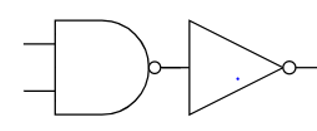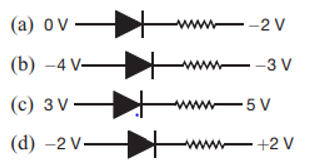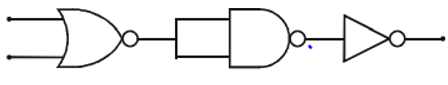# semiconductor physics class 12 mcq

Check out semiconductor physics class 12 mcq. This covers the topic like logic gates, transistor, n and p type semiconductor etc. The Types of questions are True/False, Assertion and Reason, Multiple choice questions

I hope you will enjoy this test

General Instructions

1. Your test contains multiple-choice questions with only one answer type of questions. There are a total of 20 questions
2. This is a 45 min test. Please make sure you complete it in the stipulated time
3. You can finish this test any time using the 'Submit' button.

1. When n-p-n transistor is used as an amplifier ,then
2. The forbidden energy band gaps in conductors, semiconductors, and insulators are x, y, and z respectively. The relation among them is
3. Zener diode is used for
4. At absolute zero temperature, a semi-conductor acts as a/an
5. Which of the following logic gates is a universal gate?
6. The output of OR gate is 1
7. The output of AND gate is 1
8. Drift current in a p-n junction is due to
9. if a small amount of antimony is added to the germanium crystal
10. The following diagram performs the logic function of11. You are required to choose the correct one out of the given five responses and mark it as

(a) If both assertion and reason are true and reason is the correct explanation of the assertion.

(b) If both assertion and reason are true but reason is not correct explanation of the assertion.

(c) If assertion is true, but reason is false.

(d) If both assertion and reason are false.

(e) If reason is true but assertion is false

Assertion: A transistor amplifier in common emitter configuration has a low input impedance.

Reason: The base to emitter region is forward biased

12. You are required to choose the correct one out of the given five responses and mark it as

(a) If both assertion and reason are true and reason is the correct explanation of the assertion.

(b) If both assertion and reason are true but reason is not correct explanation of the assertion.

(c) If assertion is true, but reason is false.

(d) If both assertion and reason are false.

(e) If reason is true but assertion is false

Assertion: Insulators do not allow flow of current through themselves.

Reason: They have no free charge carriers.

13. You are required to choose the correct one out of the given five responses and mark it as

(a) If both assertion and reason are true and reason is the correct explanation of the assertion.

(b) If both assertion and reason are true but reason is not correct explanation of the assertion.

(c) If assertion is true, but reason is false.

(d) If both assertion and reason are false.

(e) If reason is true but assertion is false

Assertion: A pure semiconductor has a negative temperature coefficient of resistance.

Reason: On raising the temperature, more charge carriers are released, conductance increases and resistance decreases.
14. You are required to choose the correct one out of the given five responses and mark it as

(a) If both assertion and reason are true and reason is the correct explanation of the assertion.

(b) If both assertion and reason are true but reason is not correct explanation of the assertion.

(c) If assertion is true, but reason is false.

(d) If both assertion and reason are false.

(e) If reason is true but assertion is false

Assertion: NAND is a universal gate.

Reason: It can be used to describe all other logic gates.

15. A forward biased diode is16. The circuit as shown in figure, the equivalent gate is17. The probability of electrons to be found in the conduction band of an intrinsic semiconductor at a finite temperature
18. The manifestation of band structure in solids is due to
19. If collector current is 120 mA and base current is 2 mA and resistance gain is 3, what is power gain?
20. The gate for which output is high if at least one input is low

Subscribe
Notify ofThis site uses Akismet to reduce spam. Learn how your comment data is processed.